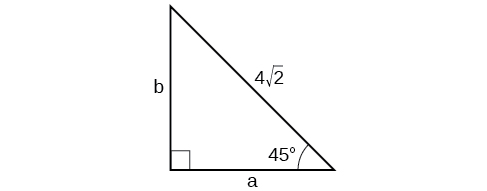$$\newcommand{\id}{\mathrm{id}}$$ $$\newcommand{\Span}{\mathrm{span}}$$ $$\newcommand{\kernel}{\mathrm{null}\,}$$ $$\newcommand{\range}{\mathrm{range}\,}$$ $$\newcommand{\RealPart}{\mathrm{Re}}$$ $$\newcommand{\ImaginaryPart}{\mathrm{Im}}$$ $$\newcommand{\Argument}{\mathrm{Arg}}$$ $$\newcommand{\norm}{\| #1 \|}$$ $$\newcommand{\inner}{\langle #1, #2 \rangle}$$ $$\newcommand{\Span}{\mathrm{span}}$$

# 5.R: Trigonometric Functions (Review)

•• Contributed by Jay Abramson
• Principal Lecturer (School of Mathematical and Statistical Sciences) at Arizona State University
• Publisher: OpenStax CNX
$$\newcommand{\vecs}{\overset { \rightharpoonup} {\mathbf{#1}} }$$ $$\newcommand{\vecd}{\overset{-\!-\!\rightharpoonup}{\vphantom{a}\smash {#1}}}$$$$\newcommand{\id}{\mathrm{id}}$$ $$\newcommand{\Span}{\mathrm{span}}$$ $$\newcommand{\kernel}{\mathrm{null}\,}$$ $$\newcommand{\range}{\mathrm{range}\,}$$ $$\newcommand{\RealPart}{\mathrm{Re}}$$ $$\newcommand{\ImaginaryPart}{\mathrm{Im}}$$ $$\newcommand{\Argument}{\mathrm{Arg}}$$ $$\newcommand{\norm}{\| #1 \|}$$ $$\newcommand{\inner}{\langle #1, #2 \rangle}$$ $$\newcommand{\Span}{\mathrm{span}}$$ $$\newcommand{\id}{\mathrm{id}}$$ $$\newcommand{\Span}{\mathrm{span}}$$ $$\newcommand{\kernel}{\mathrm{null}\,}$$ $$\newcommand{\range}{\mathrm{range}\,}$$ $$\newcommand{\RealPart}{\mathrm{Re}}$$ $$\newcommand{\ImaginaryPart}{\mathrm{Im}}$$ $$\newcommand{\Argument}{\mathrm{Arg}}$$ $$\newcommand{\norm}{\| #1 \|}$$ $$\newcommand{\inner}{\langle #1, #2 \rangle}$$ $$\newcommand{\Span}{\mathrm{span}}$$

### 5.1: Review Exercises

For the exercises 1-2, convert the angle measures to degrees.

1) $$\dfrac{π}{4}$$

$$45°$$

2) $$−\dfrac{5π}{3}$$

For the exercises 3-6, convert the angle measures to radians.

3) $$-210°$$

$$−\dfrac{7π}{6}$$

4) $$180°$$

5) Find the length of an arc in a circle of radius $$7$$ meters subtended by the central angle of $$85°$$.

$$10.385$$ meters

6) Find the area of the sector of a circle with diameter $$32$$ feet and an angle of $$\dfrac{3π}{5}$$ radians.

For the exercises 7-8, find the angle between $$0°$$ and $$360°$$ that is coterminal with the given angle.

7) $$420°$$

$$60°$$

8) $$−80°$$

For the exercises 9-10, find the angle between $$0$$ and $$2π$$ in radians that is coterminal with the given angle.

9) $$− \dfrac{20π}{11}$$

$$\dfrac{2π}{11}$$

10) $$\dfrac{14π}{5}$$

For the exercises 11-, draw the angle provided in standard position on the Cartesian plane.

11) $$-210°$$12) $$75°$$

13) $$\dfrac{5π}{4}$$14) $$−\dfrac{π}{3}$$

15) Find the linear speed of a point on the equator of the earth if the earth has a radius of $$3,960$$ miles and the earth rotates on its axis every $$24$$ hours. Express answer in miles per hour.

$$1036.73$$ miles per hour

16) A car wheel with a diameter of $$18$$ inches spins at the rate of $$10$$ revolutions per second. What is the car's speed in miles per hour?

### 5.2: Review Exercises

1) Find the exact value of $$\sin \dfrac{π}{3}$$.

$$\dfrac{\sqrt{3}}{2}$$

2) Find the exact value of $$\cos \dfrac{π}{4}$$.

3) Find the exact value of $$\cos π$$.

$$-1$$

4) State the reference angle for $$300°$$.

5) State the reference angle for $$\dfrac{3π}{4}$$.

$$\dfrac{π}{4}$$

6) Compute cosine of $$330°$$.

7) Compute sine of $$\dfrac{5π}{4}$$.

$$−\dfrac{\sqrt{2}}{2}$$

8) State the domain of the sine and cosine functions.

9) State the range of the sine and cosine functions.

$$[–1,1]$$

### 5.3: Review Exercises

For the exercises 1-4, find the exact value of the given expression.

1) $$\cos \dfrac{π}{6}$$

2) $$\tan \dfrac{π}{4}$$

$$1$$

3) $$\csc \dfrac{π}{3}$$

4) $$\sec \dfrac{π}{4}$$

$$\sqrt{2}$$

For the exercises 4-12, use reference angles to evaluate the given expression.

5) $$\sec \dfrac{11π}{3}$$

6) $$\sec 315°$$

$$\sqrt{2}$$

7) If $$\sec (t)=−2.5$$ , what is the $$\sec (−t)$$?

8) If $$\tan (t)=−0.6$$, what is the $$\tan (−t)$$?

$$0.6$$

9) If $$\tan (t)=\dfrac{1}{3}$$, find $$\tan (t−π)$$.

10) If $$\cos (t)= \dfrac{\sqrt{2}}{2}$$, find $$\sin (t+2π)$$.

$$\dfrac{\sqrt{2}}{2}$$ or $$−\dfrac{\sqrt{2}}{2}$$

11) Which trigonometric functions are even?

12) Which trigonometric functions are odd?

sine, cosecant, tangent, cotangent

### 5.4: Review Exercises

For the exercises 1-5, use side lengths to evaluate.

1) $$\cos \dfrac{π}{4}$$

2) $$\cot \dfrac{π}{3}$$

$$\dfrac{\sqrt{3}}{3}$$

3) $$\tan \dfrac{π}{6}$$

4) $$\cos (\dfrac{π}{2}) = \sin ( \_\_°)$$

$$0$$

5) $$\csc (18°)= \sec (\_\_°)$$

For the exercises 6-7, use the given information to find the lengths of the other two sides of the right triangle.

6) $$\cos B= \dfrac{3}{5}, a=6$$

$$b=8,c=10$$

7) $$\tan A = \dfrac{5}{9},b=6$$

For the exercises 8-9, use Figure below to evaluate each trigonometric function.8) $$\sin A$$

$$\dfrac{11\sqrt{157}}{157}$$

9) $$\tan B$$

For the exercises 10-11, solve for the unknown sides of the given triangle.

10)$$a=4, b=4$$

11)12) A $$15$$-ft ladder leans against a building so that the angle between the ground and the ladder is $$70°$$. How high does the ladder reach up the side of the building?

$$14.0954$$ ft

13) The angle of elevation to the top of a building in Baltimore is found to be $$4$$ degrees from the ground at a distance of $$1$$ mile from the base of the building. Using this information, find the height of the building.

## Practice Test

1) Convert $$\dfrac{5π}{6}$$ radians to degrees.

$$150°$$

2) Convert $$−620°$$ to radians.

3) Find the length of a circular arc with a radius $$12$$ centimeters subtended by the central angle of $$30°$$.

$$6.283$$ centimeters

4) Find the area of the sector with radius of $$8$$ feet and an angle of $$\dfrac{5π}{4}$$ radians.

5) Find the angle between $$0°$$ and $$360°$$ that is coterminal with $$375°$$.

$$15°$$

6) Find the angle between $$0$$ and $$2π$$ in radians that is coterminal with $$−\dfrac{4π}{7}$$.

7) Draw the angle $$315°$$ in standard position on the Cartesian plane.8) Draw the angle $$−\dfrac{π}{6}$$ in standard position on the Cartesian plane.

9) A carnival has a Ferris wheel with a diameter of $$80$$ feet. The time for the Ferris wheel to make one revolution is $$75$$ seconds. What is the linear speed in feet per second of a point on the Ferris wheel? What is the angular speed in radians per second?

$$3.351$$ feet per second, $$\dfrac{2π}{75}$$ radians per second

10) Find the exact value of $$\sin \dfrac{π}{6}$$.

11) Compute sine of $$240°$$.

$$−\dfrac{\sqrt{3}}{2}$$

12) State the domain of the sine and cosine functions.

13) State the range of the sine and cosine functions.

$$[ –1,1 ]$$

14) Find the exact value of $$\cot \dfrac{π}{4}$$.

15) Find the exact value of $$\tan \dfrac{π}{3}$$.

$$\sqrt{3}$$

16) Use reference angles to evaluate $$\csc \dfrac{7π}{4}$$.

17) Use reference angles to evaluate $$\tan 210°$$.

$$\dfrac{\sqrt{3}}{3}$$

18) If $$\csc t=0.68$$, what is the $$\csc (−t)$$?

19) If $$\cos t= \dfrac{\sqrt{3}}{2}$$, find $$\cos (t−2π)$$.

$$\dfrac{\sqrt{3}}{2}$$

20) Which trigonometric functions are even?

21) Find the missing angle: $$\cos \left(\dfrac{\pi }{6} \right)= \sin (\;)$$

$$\dfrac{π}{3}$$

22) Find the missing sides of the triangle $$ABC: \sin B= \dfrac{3}{4},c=12$$

23) Find the missing sides of the triangle.$$a=\dfrac{9}{2},b=\dfrac{9\sqrt{3}}{2}$$
24) The angle of elevation to the top of a building in Chicago is found to be $$9$$ degrees from the ground at a distance of $$2000$$ feet from the base of the building. Using this information, find the height of the building.